Windowenergeto 8000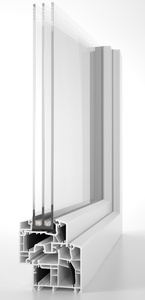Component id: 1091ws04 aluplast Ibérica S.L.U. Window System PVC 1.00 W/(m2 K) phC SWISSPACER Ultimate Warm, temperate At some not installed frame sections the temperature factor is not achieved. That might leed to hygienic subotimal conditions in case of very low exterior temperatures.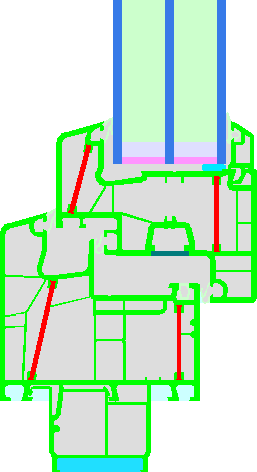Frame cut Frame width
bf/mm
Frame U value
Uf/(W/(m2 K))
Glass edge Ψ value
Ψg/(W/(m K))
Temperature factor
fRsi = 0,25 m2K/W
Threshold side entrance 89 1.63 0.022 0.42
Threshold balcony 82 1.48 0.023 0.46
Bottom 148 1.12 0.024 0.68
Bottom (Variant 1) 119 0.96 0.024 0.71
Transom 2 casements 181 1.06 0.023 0.67
Transom Fixed 104 1 0.023 0.72
Top Fixed 80 0.83 0.023 0.73
Bottom Fixed 110 1.08 0.023 0.69
Lateral Fixed 80 0.83 0.023 0.73
Transom 1 casement 143 1.04 0.023 0.68
Lateral 119 0.96 0.024 0.71
Flying Mullion thick 162 1.00 0.023 0.62
Flying Mullion narrow 142 1.05 0.022 0.62
Mullion Fixed 104 1 0.023 0.72
Mullion 2 casements 181 1.06 0.023 0.67
Mullion 1 casement 143 1.04 0.023 0.68
Top 119 0.96 0.024 0.71

Please select preferred window-layout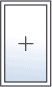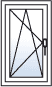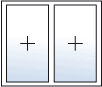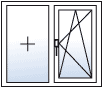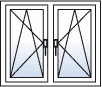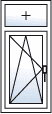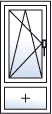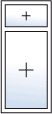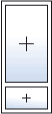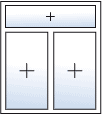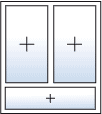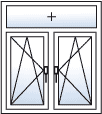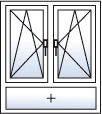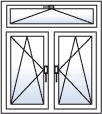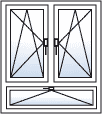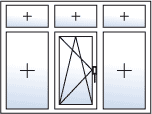U-Value Calculation

 b1 =  m b2 =  m b3 =  m htop =  m hbottom =  mGlazing Standard (Ug = 0.7) arcon N solar (Ug = 0.6) arcon N solar (Ug = 0.69) arcon N solar (Ug = 0.64) arcon N solar (Ug = 0.77) arcon N solar (Ug = 0.67) arcon N solar (Ug = 0.75) ClimaGuard nrG (Ug = 0.6) ClimaGuard nrG (Ug = 0.69) ClimaGuard nrG (Ug = 0.75) ClimaGuard nrG (Ug = 0.64) ClimaGuard Premium (Ug = 0.49) ClimaGuard Premium (Ug = 0.64) ClimaGuard Premium2 (Ug = 0.64) ClimaGuard Premium2 (Ug = 0.49) iplus 3 CE (Ug = 0.49) iplus 3 CE (Ug = 0.56) iplus 3 CLS und CLST (Ug = 0.6) iplus 3 CLS und CLST (Ug = 0.67) iplus 3 E (Ug = 0.58) iplus 3 E (Ug = 0.64) iplus 3 E (Ug = 0.72) iplus 3 LS und LST (Ug = 0.75) iplus 3 LS und LST (Ug = 0.69) iplus 3 LS und LST helles float (Ug = 0.75) iplus 3 LS und LST helles float (Ug = 0.69) iplus Advanced 1.0 (Ug = 0.51) iplus Advanced 1.0 (Ug = 0.59) iplus Top 1.1 (Ug = 0.58) iplus Top 1.1 (Ug = 0.49) iplus Top 1.1 (Ug = 0.64) iplus Top 1.1 (Ug = 0.61) iplus Top 1.1 (Ug = 0.56) iplus Top 1.1T (Ug = 0.49) iplus Top 1.1T (Ug = 0.58) iplus Top 1.1T (Ug = 0.56) iplus Top 1.1T (Ug = 0.61) iplus Top 1.1T (Ug = 0.64) Passiv-Therm (Ug = 0.6) Planibel Tri (Ug = 0.73) Planibel Tri (Ug = 0.67) Planibel Tri (Ug = 0.75) Planibel Tri (Ug = 0.62) Planibel Tri (Ug = 0.65) Planibel Tri (Ug = 0.58) RX WARM (Ug = 0.56) RX WARM (Ug = 0.64) RX WARM (Ug = 0.72) RX WARM (Ug = 0.49) RX WARM (Ug = 0.66) RX WARM (Ug = 0.58) Solar-Therm (Ug = 0.71) Super-Therm (Ug = 0.79) TOP Premium 0.6 (Ug = 0.58) TOP Premium E 0.6 (Ug = 0.58) TOP Solar 0.7 (Ug = 0.69) Ultra-Therm (Ug = 0.6) VITAL 0.6 (Ug = 0.6) VITAL 0.6 (Ug = 0.66) VITAL 0.6 (Ug = 0.55) VITAL 0.7 (Ug = 0.69) VITAL 0.7 (Ug = 0.64) VITAL 0.7 (Ug = 0.75) SGG CLIMATOP ECLAZ ONE & ONE II (Ug = 0.67) EAGON SUPER VIG (Ug = 0.51) ClimaGuard Premium2 (Ug = 0.53) ClimaGuard Premium2 (Ug = 0.58) SGG CLIMATOP PLANITHERM ONE & ONE II (Ug = 0.6) SGG CLIMATOP PLANITHERM XN & XN II (Ug = 0.71) SGG CLIMATOP PLANITHERM LUX & LUX II (Ug = 0.79) SGG CLIMATOP PLANITHERM LUX & LUX II (Ug = 0.63) SGG CLIMATOP PLANITHERM LUX & LUX II (Ug = 0.72) SGG CLIMATOP PLANITHERM LUX & LUX II (Ug = 0.66) SGG CLIMATOP PLANITHERM XN & XN II (Ug = 0.64) EAGON SUPER VIG Plus_(air) (Ug = 0.47) SGG CLIMATOP PLANITHERM XN & XN II (Ug = 0.58) EAGON SUPER VIG Plus_Ar (Ug = 0.34) SGG CLIMATOP PLANITHERM XN & XN II (Ug = 0.55) EAGON SUPER VIG Plus_Kr (Ug = 0.33) SGG CLIMATOP ECLAZ & ECLAZ II (Ug = 0.55) SGG CLIMATOP PLANITHERM ONE & ONE II (Ug = 0.54) SGG CLIMATOP PLANITHERM ONE & ONE II (Ug = 0.5) SGG CLIMATOP ECLAZ & ECLAZ II (Ug = 0.71) iplus Advanced 1.0 (Ug = 0.53) iplus Advanced 1.0 (Ug = 0.56) iplus Advanced 1.0 (Ug = 0.44) SGG CLIMATOP ECLAZ & ECLAZ II (Ug = 0.64) SGG CLIMATOP ECLAZ & ECLAZ II (Ug = 0.58) SGG CLIMATOP ECLAZ ONE & ONE II (Ug = 0.5) SGG CLIMATOP ECLAZ ONE & ONE II (Ug = 0.54) SGG CLIMATOP ECLAZ ONE & ONE II (Ug = 0.6) iplus 3 (Ug = 0.58) iplus 3 (Ug = 0.72) iplus 3 (Ug = 0.64) iplus 3C (Ug = 0.56) iplus 3C (Ug = 0.49) iplus 3CLS (Ug = 0.6) iplus 3LS (Ug = 0.74) iplus 3CLS (Ug = 0.67) iplus 3LS (Ug = 0.69) Ug  W/(m2 K) G-Value UW = ? W/(m2 K)

Installationsituation

UW, installed = ? W/(m2 K)

Cost Calculation

Orientation :

Please choose orientation of the window
to get the PHPP-standard values for solar radiation

kKh/a

kWh/(m2a)

Recommended Value for Reduction Factor is 0.9
Shading Factor should be between 0.1 and 1. For standard situations look in ISO EN 13790

€/kWh

%

Years

Present Value(energy) = ? €
Present Value(total) = ? €
Back to list